## Learn about some canculator formulas we have available in Ion!

Ion's rule structure uses NCalc to perform its calculations. Variables in NCalc formulas can be replaced with dynamic data fields in Ion in order to allow users to insert their own values and render a calculation based on those values. Outlined below are some of the most common use cases for NCalc formulas, as well as a table featuring all available NCalc formulas.

Before attempting any of the formulas below, we recommend that you have gone through our calculator trainings or have some background working with calculations in Ion as these formulas serve very advanced and specific purposes.

### Exponent (Power)

The exponent (power) formula returns the user's value raised by a specified amount. The exponent formula "pow(base,exponent)" requires two values separated by a comma. It's possible to make both of those values dynamic if necessary: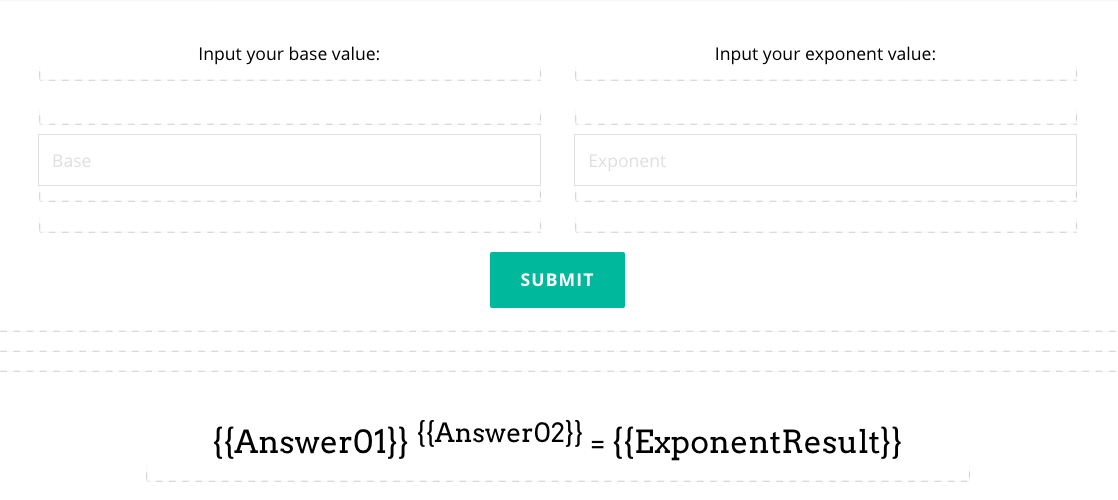In this example, the page contains two form inputs: one to capture the Base value, and one to capture the Exponent value.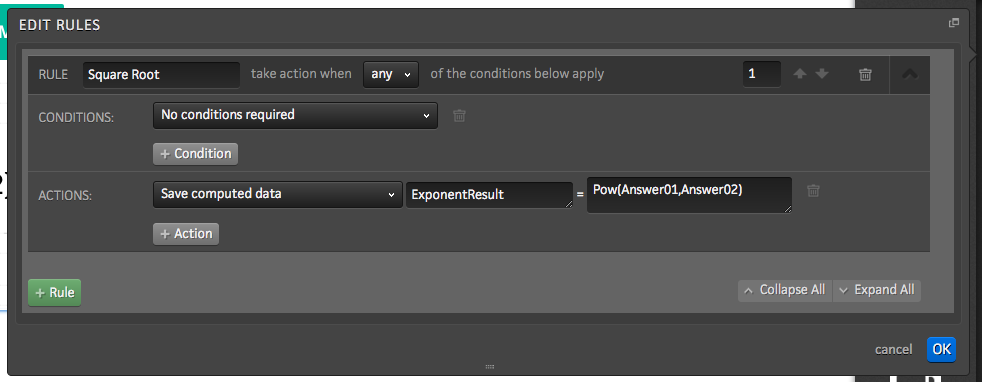Once the user submits the two values, the page refreshes and runs the rule displayed above. In this rule, Answer01 is raised to the power of Answer02 and saved into a new data field called "ExponentResult".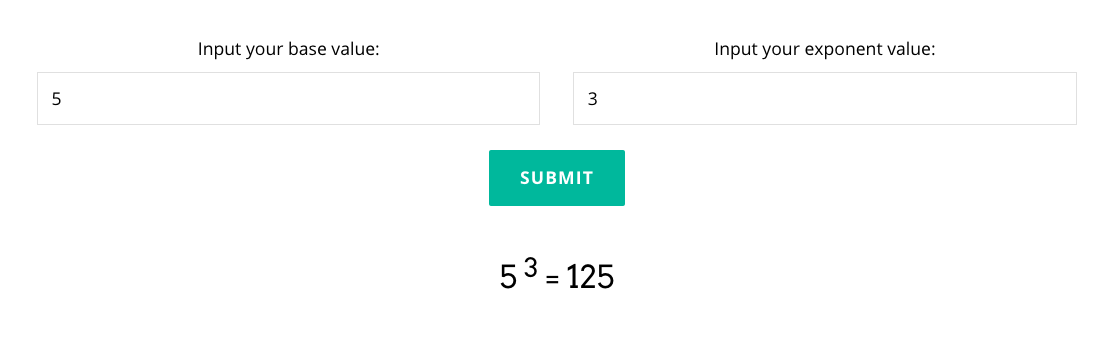On the page, the dynamic text content ("=") is replaced with the user submitted values and the calculated ExponentResult.

### Round

The round formula "Round(Number, Decimals)" allows users to round any number to a particular amount of decimal places. This formula is more commonly used to round a number generated in calculations.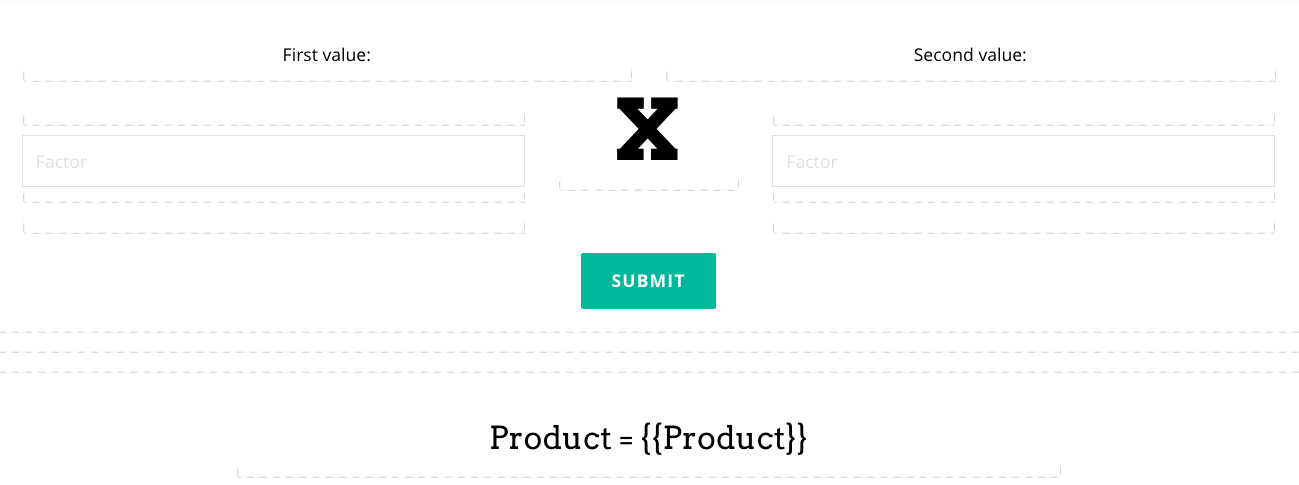In the example above, the user is able to input two values, which save to data fields "Answer01" and "Answer02".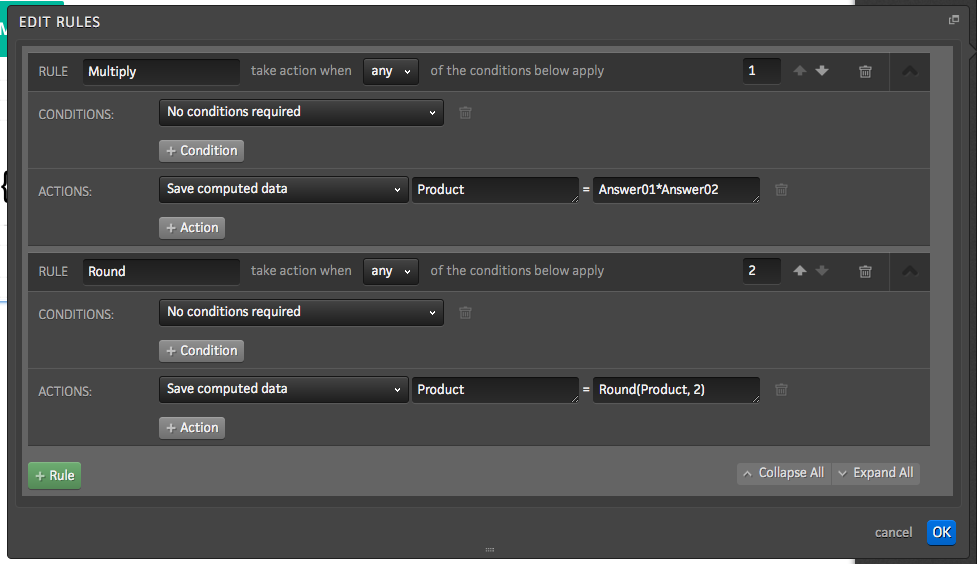On submit, the page refreshes and runs two rules. The first simply multiplies the two factor variables by one another and saves them into the new data field "Product". The second uses the Round formula to round the Product result by 2 decimals and updates the Product data field with the new rounded value.The final Product should equal "42.59025", but because there is a second rule in place to round the value down to the nearest two decimal places, the result is just "42.59", instead.

### Logarithm

The "Log(Number, Base)" formula returns the logarithm for the user's input. The base can be dynamic and controlled by the user, or entered into the calculation as a constant (ie. a base of "10" to calculate the common logarithm).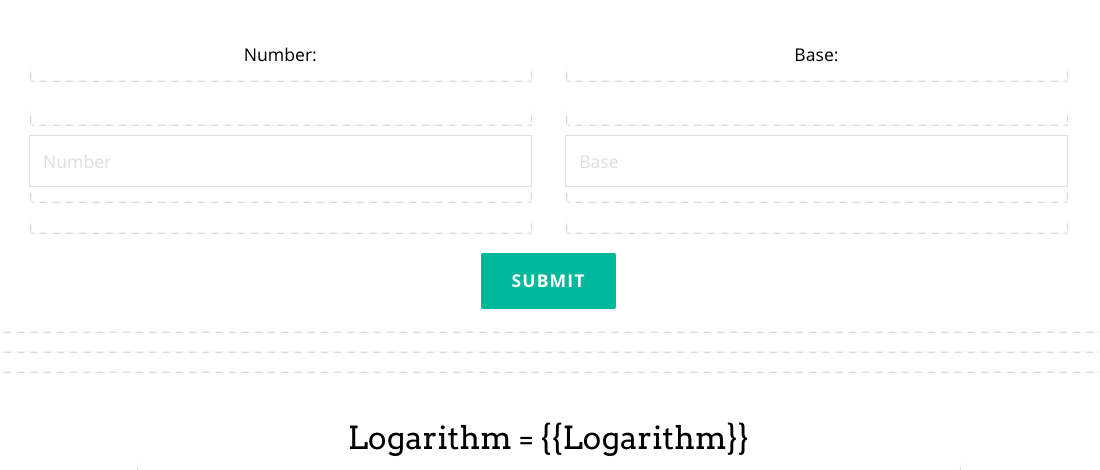In the example above, the user is able to input two values, the first for the number and the second as the base, which save to data fields "Answer01" and "Answer02".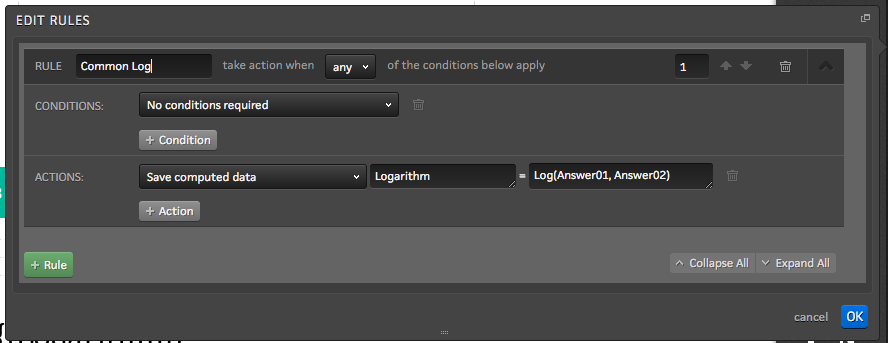This rule computes the logarithm of the number Answer01 by the base Answer02 and saves the value into a new data field called "Logarithm".Once the rule has run, the dynamic content variable on the page (originally "Logarithm = ") is updated to display the computed value.

### Natural Logarithm

The Natural Logarithm can also be computed using a combination of two NCalc formulas "Log(Number, Exp(1))", where Number is the user submitted variable and Exp(1) returns the value of e.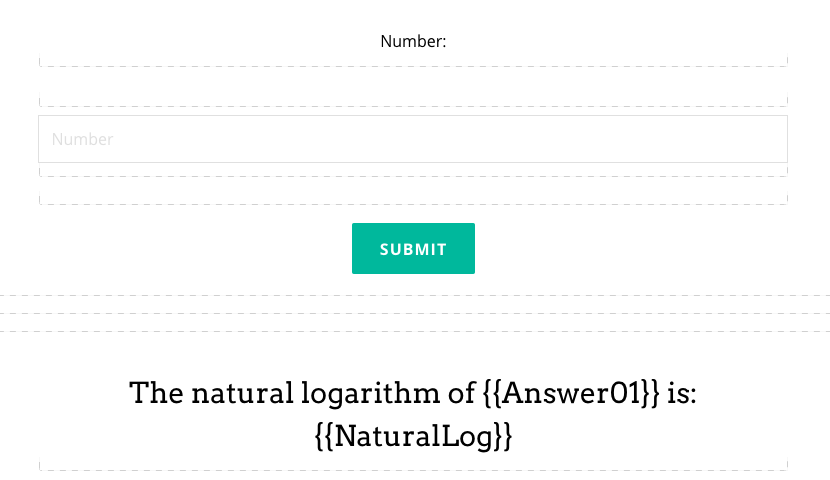In the example above, the user is able to input a value which save to the "Answer01" data field.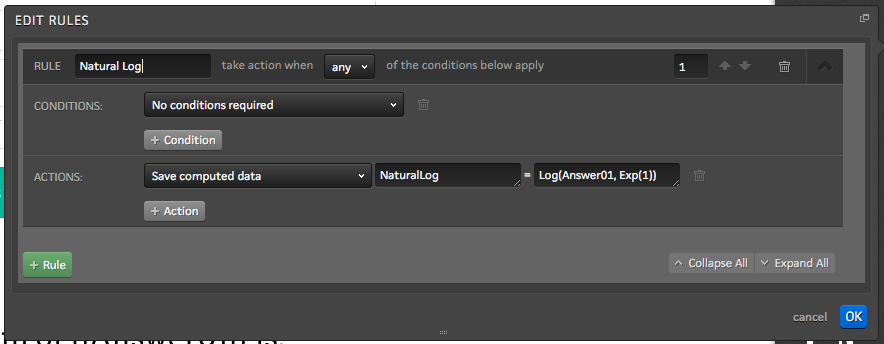This rule computes the Log of Answer01 with a base of e. It then saves that value into a new data field called "NaturalLog".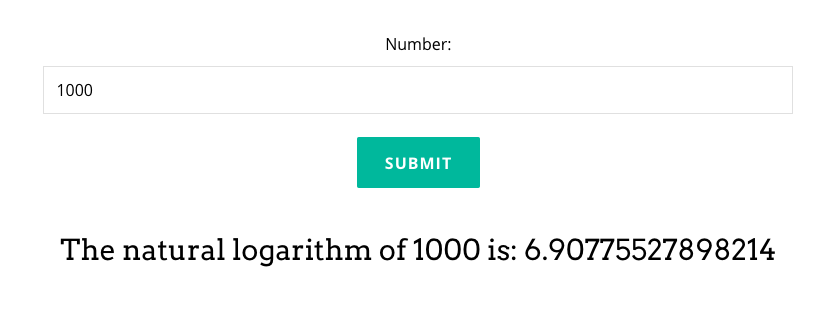Once the rule has run, the dynamic content variable on the page (originally "The natural logarithm of 1000 is: ") is updated to display the computed value.

### Square Root

The square root formula "sqrt( )" returns the square root of the value in the parenthesis. If a user were to submit a value into a data field, a rule could be configured to find "sqrt(UserValue)" and save the value into a new data field (in this example, "SquareRoot"). In the example below, the user input is saved as "Answer01".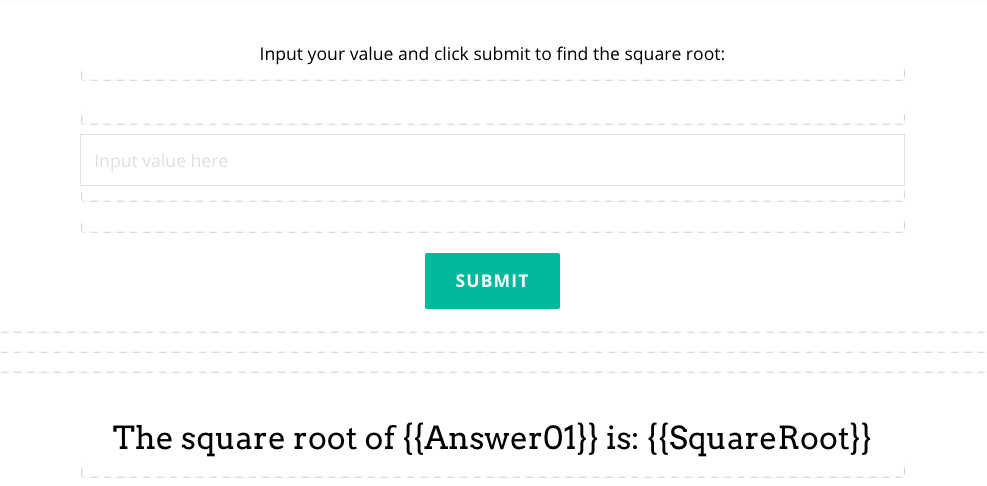The form contains one input saved into data field "Answer01".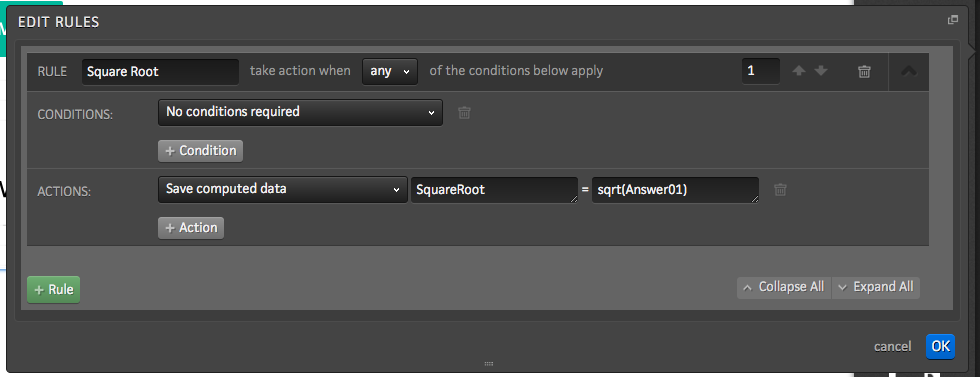When the user inputs a value and clicks submit, the page refreshes and runs the rule displayed above. The square root of Answer01 is saved into a new data field, in this instance, called "SquareRoot".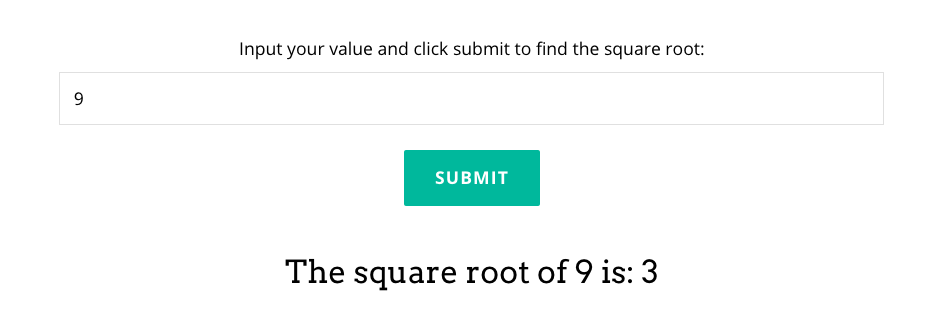Once the run has run, the dynamic content variables on the page (originally "The square root of is: ") are updated to display both the user's input and the computed value.

All Available NCalc Formulas

 Name Description Usage Result Abs Returns the absolute value of a specified number. Abs(-1) 1M Acos Returns the angle whose cosine is the specified number. Acos(1) 0d Asin Returns the angle whose sine is the specified number. Asin(0) 0d Atan Returns the angle whose tangent is the specified number. Atan(0) 0d Ceiling Returns the smallest integer greater than or equal to the specified number. Ceiling(1.5) 2d Cos Returns the cosine of the specified angle. Cos(0) 1d Exp Returns e raised to the specified power. Exp(0) 1d Floor Returns the largest integer less than or equal to the specified number. Floor(1.5) 1d IEEERemainder Returns the remainder resulting from the division of a specified number by another specified number. IEEERemainder(3, 2) -1d Log Returns the logarithm of a specified number. Log(1, 10) 0d Log10 Returns the base 10 logarithm of a specified number. Log10(1) 0d Max Returns the larger of two specified numbers. Max(1, 2) 2 Min Returns the smaller of two numbers. Min(1, 2) 1 Pow Returns a specified number raised to the specified power. Pow(3, 2) 9d Round Rounds a value to the nearest integer or specified number of decimal places. The mid number behavior can be changed by using EvaluateOption.RoundAwayFromZero during construction of the Expression object. Round(3.222, 2) 3.22d Sign Returns a value indicating the sign of a number. Sign(-10) -1 Sin Returns the sine of the specified angle. Sin(0) 0d Sqrt Returns the square root of a specified number. Sqrt(4) 2d Tan Returns the tangent of the specified angle. Tan(0) 0d Truncate Calculates the integral part of a number. Truncate(1.7) 1